# Communications - Online Test

Q1. During transmission over a certain binary communication channel, bit errors occur independently with probability p. The probability of AT MOST one bit in error in a block of n bits is given by
Explaination / Solution: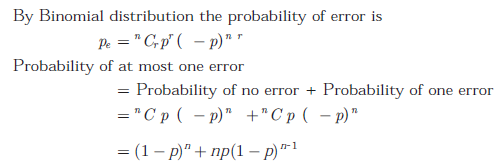Q2. Transducer is a device that
Explaination / Solution:

Any device which converts energy from one form to another is called a transducer.It converts variation in physical quantities such as pressure,dispalcement,force,temprature etc.Like microphone converts sound signal into an electrical signal.

Q3. In a GSM system, 8 channels can co-exist in 200 kHz bandwidth using TDMA. A GSM based cellular operator is allocated 5 MHz bandwidth. Assuming a frequency reuse factor of 1/5, i.e. a five-cell repeat pattern, the maximum number of simultaneous channels that can exist in one cell is
Explaination / Solution: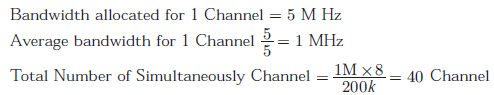Q4. Which of these can be used to convert position signals to electrical signals?
Explaination / Solution:

Potentiometer is device which can be used to measure and control frequency,temprature etc.Hence changing frequency in signal coverts it into electrical signal.

Q5. In a Direct Sequence CDMA system the chip rate is 1.2288 × 106 chips per second. If the processing gain is desired to be AT LEAST 100, the data rate
Explaination / Solution:Q6. Which of these is an acoustic transducer?
Explaination / Solution:

Acoustic transducer is a device which converts one form of signal into another without any direct contact.

Q7. An air-filled rectangular waveguide has inner dimensions of 3 cm × 2 cm. The wave impedance of the TEmode of propagation in the waveguide at a frequency of 30 GHz is (free space impedance η0 = 377Ω)
Explaination / Solution: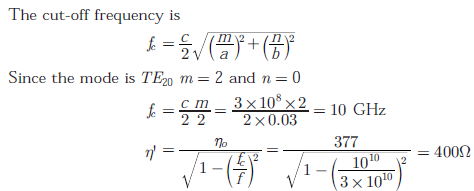Q8. Digital weighing balance uses
Explaination / Solution:

A force transducer is also known as a force sensor this converts an input mechanical force into output electrical signal.Hence a force transducer is used in digital weighing balance.

Q9. The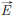field in a rectangular waveguide of inner dimension a × b is given byWhere H0 is a constant, and a and b are the dimensions along the x − axis and the y − axis respectively. The mode of propagation in the waveguide is
Explaination / Solution: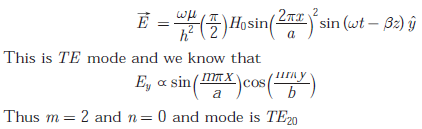Q10. Signal in electronic communication refers to
Explaination / Solution:

In communication a signal implies a time varying electrical signal obtained by transforming original signal with the help of a suitable transducer.It is single valued function of time that conveys the information.